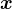A probability distribution gives the probability of a random variable being (exactly|in_the_range_of) some value.

## Expected Value

The expected value ⟨X⟩ of a random variable X is the mean, the average, the value of the argument you have to supply to the probability distribution to get the maximum of the function.where f is the probability mass function.

Or for continuous distributions:where ϕ is the probability density function.

## Variance

The variance V, given some data points, is:Sometimes, the variance of the entire set (not just the sample) is:where n is the maximum index of.